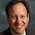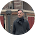## Monday, January 14, 2013

### For loops (and how to avoid them)

My experience when starting out in R was trying to clean and recode data using for() loops, usually with a few if() statements in the loop as well, and finding the whole thing complicated and frustrating.

In this post, I'll go over how you can avoid for() loops for both improving the quality and speed of your programming, as well as your sanity.

So here we have our classic dataset called mydata.Rdata (you can download this if you want, link at the right):

And if I were in Stata and wanted to create an age group variable, I could just do:

gen Agegroup=1
replace Agegroup=2 if Age>10 & Age<20
replace Agegroup=3 if Age>=20

But when I try this in R, it fails:Why does it fail? It fails because Age is a vector so the condition if(mydata\$Age<10) is asking "is the vector Age less than 10", which is not what we want to know.  We want to ask, row by row is each element of Age<10, so we need to specify the element of the vector we're referring to. We don't specify the element and thus we get the warning (really, error), "only the first element will be used."  So when this fails, the first way people try to solve this problem is with a crazy for() loop like this:

###########Unnecessarily long and ugly code below#######
mydata\$Agegroup1<-0

for (i in  1:10){
if(mydata\$Age[i]>10 & mydata\$Age[i]<20){
mydata\$Agegroup1[i]<-1
}
if(mydata\$Age[i]>=20){
mydata\$Agegroup1[i]<-2
}
}

Here we tell R to go down the rows from i=1 to i=10, and for each of those rows indexed by i, check to see what value of Age it is, and then assign Agegroup a value of 1 or 2.  This works, but at a high cost - you can easily make a mistake with all those indexed vectors, and also for() loops take a lot of computing time, which would be a big deal if this dataset were 10000 observations instead of 10.

So how can we avoid doing this?

One of the most useful functions I have found is one that I have referred to a number of times in my blog so far - the ifelse() function.  The ifelse() function evaluates a condition, and then assigns a value if it's true and a value if it's false.  The great part about it is that it can read in a vector and check each element of the vector one by one so you don't need indices or a loop. You don't even need to initialize some new variable before you run the statement.  Like this:

mydata\$newvariable<-ifelse(Condition of some variable,
Value of new variable if condition is true
Value of new variable if condition is false)

so for example:

mydata\$Old<-ifelse(mydata\$Age>40,1,0)

This says, check to see if the elements of the vector mydata\$Age are greater than 40: if an element is greater than 40, it assigns the value of 1 to mydata\$Old, and if it's not greater than 40, it assigns the value of 0 to mydata\$Old.

But we wanted to assign values 0, 1, and 2 to an Agegroup variable.  To do this, we can use nested ifelse() statements:

mydata\$Agegroup2<-ifelse(mydata\$Age>10 & mydata\$Age<20,1,
ifelse(mydata\$Age>20, 2,0))

Now this says, first check whether each element of the Age vector is >10 and <20.  If it is, assign 1 to Agegroup2.  If it's not, then evaluate the next ifelse() statement, whether Age>20.  If it is, assign Agegroup2 a value of 2.  If it's not any of those, then assign it 0.  We can see that both the loop and the ifelse() statements give us the same result:

You can nest ifelse() statement as much as you like. Just be careful about your final category - it assigns the last value to whatever values are left over that didn't meet any condition (including if a value is NA!) so make sure you want that to happen.

Other examples of ways to use the ifelse() function:
• If you want to add a column with the mean of Weight by sex for each individual, you can do this with ifelse() like this:
mydata\$meanweight.bysex<-ifelse(mydata\$Sex==0,
mean(mydata\$Weight[mydata\$Sex==0], na.rm=TRUE),
mean(mydata\$Weight[mydata\$Sex==1], na.rm=TRUE))

• If you want to recode missing values:
mydata\$Height.recode<-ifelse(is.na(mydata\$Height),
9999,
mydata\$Height)

• If you want to combine two variables together into a new one, such as to create a new ID variable based on year (which I added to this dataframe) and ID:
mydata\$ID.long<-ifelse(mydata\$ID<10,
paste(mydata\$year, "-0",mydata\$ID,sep=""),
paste(mydata\$year, "-", mydata\$ID, sep=""))

Other ways to avoid the for loop:

• The apply functions:  If you think you have to use a loop because you have to apply some sort of function to each observation in your data, think again! Use the apply() functions instead.  For example:
• You can also use other functions such as cut() to do the age grouping above. Here's the post on how this function works, so I won't go over it again, except to say if you convert from a factor to a numeric, *always* convert to a character before converting it to numeric:
mydata\$Agegroup3<-as.numeric(as.character(cut(mydata\$Age, c(0,10,20,100),labels=0:2)))

Basically, any time you think you have to do a loop, think about how you can do it with another function. It will save you a lot of time and mistakes in your code.

1.Also possible:
attach(mydata)
Agegroup[Age < 10] <- 2

Cheers, Andrej

1.2.Okay... so I am trying to to write a loop that looks for column values <0 in columns 1 and 2 and then prints the row names where this is the case... I have tried for the last day various different things with and without for loops and am getting no where, any ideas?

Thanks!

Millie

1.Hmm, well you can do this:

##create some data
data1<-as.data.frame(cbind(c(1,-2,-3,0,-5),c(-1,-2,3,0,0)))
colnames(data1)<-c("col1","col2")

##print out rownames of data1 where either column 1 or 2 is <0
rownames(data1)[data1\$col1<0 | data1\$col2<0]

Is that what you're going for?

2.That was exactly what I was going for! THANK YOU!!! :)

3.:) No problem, glad it works!

3.mydata\$Agegroup[which(mydata\$Age <= 10)] <- 1
works too

4.I strongly recommend that you look at a package called data.table. The syntax is quite simple and the speed is just out of this world.

5.Great, thanks everyone. Another user recommended the recode function which looks great. I'll do an update post on these suggestions soon.

6.Thank you, thats very interesting information. I need to share with my friends.

back muscle pain

7.Excellent posting. Many people will like it. Thank you so much!

Latisse generic

8.Thanks so much!

9.I'm doing linear regression model and I need to use the predict() function to get the prob., however there are too many category under each variable. Are there any function that I can use to let R run the predict() function for me instead of copy-and-paste? Thank you in advance. :)

10.This comment has been removed by the author.

11.ifelse is great but a nightmare when you have, say 17 nested conditions:

ifelse(,, ifelse(,, ifelse(,, ifelse(,, ifelse(,, ifelse(,, ifelse(,, ifelse(,, ifelse(,, ifelse(,, ifelse(,, ifelse(,, ifelse(,, ifelse(,, ifelse(,, ifelse(,, ifelse(,,)))))))))))))))))

Note: Only a member of this blog may post a comment.